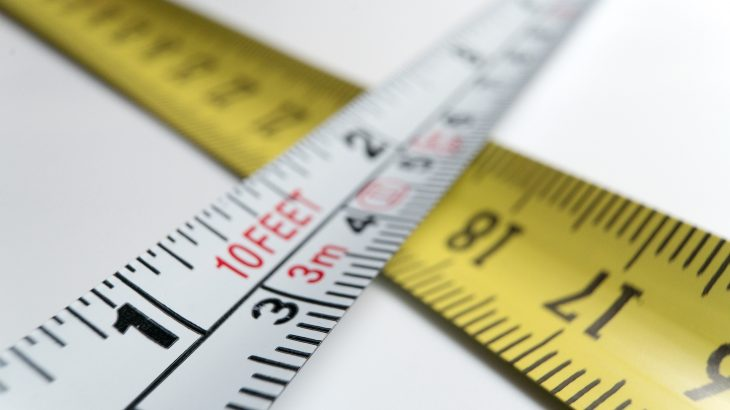# Measurement unit conversion: US vs Metric & Japan unitsQuickly convert US measurement units such as inches, feet, pounds, and gallon in to metric or unique Japanese measurement units.

#### Length / 長さ

US Metric
1” (inch) 25.4 mm
1’ (foot) = 12” 30.48 cm = 304.8 mm
1 yard = 3’ = 36” 0.91 m = 91.44 cm
1 mile = 1760 yard = 5280’ 1.6 km = 1609 m
0.62 miles 1 km = 1000 m
1.09 yards = 3.28’ = 39.37” 1 m = 100 cm = 1000 mm
1 mm = 1000 μm = 1,000,000 nm

#### Mass / 重さ

US Metric
1 oz (Ounce) 28.35 g
1 lb (Pound) = 16 oz 0.453 kg = 453.59 g
1 Stone = 14 lbs = 224 oz 6.35 kg
1 US ton = 2000 lbs = 0.893 UK ton 0.907 Metric ton = 907.19 kg
1.102 US ton = 2204.62 lbs 1 Metric ton = 1000 kg
2.20 lbs = 35.27 oz 1 kg = 1000 g
1 g = 1,000 mg = 1,000,000 μg

#### Area / 面積

US Metric & Japanese
1 Foot2 = 144 Inch2 0.0929 m2 = 929.03 cm2
1 Yard2 = 9 Foot2 0.836 m2 = 8361.3 cm2
1 Acre = 4840 Yard2 = 43560 Foot2 (=660’x66’) 0.405 ha = 4046.86 m2 = 1226 Tsubo (坪)
1 mile2 = 640 Acre 2.59 km2 = 259 ha (hectare)
0.386 mile2 = 247.1 Acre 1 km2 = 100 ha
10.76 Foot2 1 m2 = 10000 cm2
35.58 Foot2 1 JP Tsubo (坪) = 3.31 m2

#### Volume / 体積

US Metric & Japanese
1 US Teaspoon = 0.83 UK Teaspoon 4.93 mL (cc) = 0.99 JP Ko-saji (小さじ)
1 US Tablespoon = 3 US Teaspoon 14.79 mL = 0.99 JP Oh-saji (大さじ)
1 US Fl.oz (液量オンス) = 2 US Tablespoon 29.57 mL
1 US Legal (規格計量) cup = 8.12 US Fl. oz 240 mL = 1.20 JP cup
1 US Customary (慣用) cup = 8 US Fl.oz 236.59 mL
1 US Pint = 16 US Fl.oz = 0.83 UK Pt 473.18 mL
1 US Quart = 2 US Pt = 0.83 UK Qrt 0.95 L = 946.35 mL
1 US Gallon = 4 US Qrt = 0.83 UK Gal 3.79 L = 3785.41 mL
1 Foot3 = 7.48 Gal = 1728 Inch3 28.32 L
35.31 Foot3 = 264.17 Gal 1 m3 = 1000 L
0.26 Gal = 1.06 Qrt. = 33.81 Fl.oz 1 L = 1000 mL
0.83 US Legal cup 1 JP cup = 200 mL
1.014 US Tablespoon = 0.51 Fl.oz 1 JP Oh-saji (大さじ) = 3 JP Ko-saji = 15 mL
1.014 US Teaspoon = 0.17 Fl.oz 1 JP Ko-saji (小さじ) = 5 mL
0.48 Gal = 60.997 Fl.oz 1 JP Sho (一升) = 10 JP Go = 1.804 L
6.10 Fl.oz 1 JP Go (合) = 180.39 mL

## Freelance Japanese Interpreters, On-DemandFind a Japanese interpreter,
in San Francisco, New York, or anywhere in the US.

• 2018.12.13

• 2018.11.19

• 2018.11.19